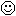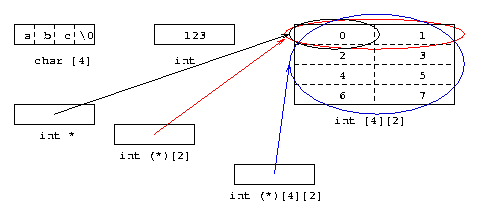## More Words about Arrays and Pointers

As noted elsewhere, C has a very important rule about arrays and pointers.  This rule -- The Rule -- says that, in a value context, an object of type ‘array of T’ becomes a value of type ‘pointer to T’, pointing to the first element of that array.  Because the result is a value, not an object, The Rule only applies once to any particular sub-expression.  If the pointer value -- of type ‘pointer to T’, that is -- itself points to another array, however, the result of indirecting through that pointer is again an array object, and The Rule may (or may not) apply again (depending on whether that object is ‘in a value context’).

By far the best way to understand all this is to examine a diagram of several actual objects.  Here is one such diagram, in which our C system has at least six objects.  One of these objects is an array of four chars, or as I prefer to say, has type ‘array 4 of char’.  This array holds a string, "abc".  Another is an ordinary int that is set to 123.  These two objects are just floating around uselessly in this diagram so as to clutter it up.They are the same size, suggesting that this implementation has sizeof(int)==4.

The remaining four objects are the important ones.  The largest box (in black) is an object of type ‘array 4 of array 2 of int’, or int .  Its elements are initialized to values counting up from 0 to 7.  There are also three pointers, and each one has a different type.This particular diagram might result from entering a function that looks like this:
```void f(void) {
int matrix = { {0,1}, {2,3}, {4,5}, {6,7} };
char s[] = "abc";
int i = 123;
int *p1 = &matrix;
int (*p2) = &matrix;
int (*p3) = &matrix;
/* code goes here */
}
```
The differences between the pointers are not so much ‘where they point’ as, in this illustration, how much they point-to.  All three pointers certainly allow you to locate the 0 in matrix, and if you convert these pointers to ‘byte addresses’ and print them out with a %p directive in printf(), all three are quite likely to produce the same output (on a typical modern computer).  But the int * pointer, p1, points only to a single int, as circled in black.  The red pointer, p2, whose type is int (*), points to two ints, and the blue pointer -- the one that points to the entire matrix -- really does point to the entire matrix.

These differences affect the results of both pointer arithmetic and the unary * (indirection) operator.  Since p1 points to a single int, p1 + 1 moves forward by a single int.  The black circle is only as big as one int, and *(p1 + 1) is just the next int, whose value is 1.  Likewise, sizeof *p1 is just sizeof(int) (probably 4).

Since p2 points to an entire ‘array 2 of int’, however, p2 + 1 will move forward by one such array.  The result would be a pointer pointing to a red circle going around the {2,3} pair.  Since the result of an indirection operator is an object, *(p2 + 1) is that entire array object, which may fall under The Rule.  If it does fall under The Rule, the object will become instead a pointer to its first element, i.e., the int currently holding 2.  If it does not fall under The Rule -- for instance, in sizeof *(p2 + 1), which puts the object in object context -- it will remain the entire array object.  This means that sizeof *(p2 + 1) (and sizeof *p2 as well, of course) is sizeof(int) (probably 8).

Finally, p3 points to the whole matrix, and p3 + 1 will move forward by one whole matrix.  This would be a blue circle around something that is not even there.  ANSI C allows you to calculate such a pointer, but not to use it with an indirection .  Since *(p3 + 1) is itself an error, it is probably best just to note that *p3 is the entire matrix, and thus sizeof *p3 is sizeof(int ), or 4*2*sizeof(int) -- in this case, most likely 32 (since sizeof(int) looks like 4 in the diagram above).

 Actually, it is really an ellipse.  Picky, picky.
 Most implementations handle this with no trouble at all, as most implementations have little or no pointer checking.  Those that do check might need to allocate an extra byte or word after each object -- or more efficiently, after ‘the last’ object in a clump of objects -- just so that there is a place for a ‘one beyond the last’ pointer to point-to.